Tweeter Mids Fullrange Woofer no longer available PA Mids Woofer Fullrange no longer available Car Woofer no longer available

# Monacor

Monacor develops and produces products for HiFi, Car HiFi, PA, Multimedia and Home Cinema since 1965. The brands like MONACOR, IMG Stage Line and CARPOWER are well known for their high quality.

## 12 inch wooferSP-302e       order no. mo-104000       EUR 72,45excl. VAT: € 60.88 / \$ 65.75
29 cm bass driver with high quality cone.
• power handling (continuous/programme) = 100/200 W
• frequency range = 40-5500 Hz
• resonance frequency fs = 39 Hz
• impedance R = 4 Ohm
• sound pressure level SPL = 95 dB (2,83V; 1m)
• mounting diameter d = 281 mm
• overall diameter d = 304 mm
• mounting depth (not countersunk) t = 114 mmrecommended cabinet 1:
closed cabinet with 20 L volume
from 109/69 Hz (-3dB/-8dB)

recommended cabinet 2:
63 L volume bass reflex cabinet
with 2x HP100 reflex tube, 13 cm long.
from 58/45 Hz (-3dB/-8dB).

SP-30A/200PRO       order no. mo-104520       - not available (_)
29 cm bass driver with high quality cone. The basket is made of die-cast.
• power handling (continuous/programme) = 200/400 W
• frequency range = 30-3000 Hz
• resonance frequency fs = 45 Hz
• impedance R = 8 Ohm
• sound pressure level SPL = 102 dB (2,83V; 1m)
• DC resistance Re = 5,8 Ohm
• force factor BL = 13,95 N/A
• voice coil inductance L = 0,7 mH
• effective piston radiating area Sd = 530 cm2
• effective mechanical mass incl. air load mms = 43 g
• equivalent volume of compliance Vas = 114 l
• total Q factor Qts = 0,33 (Qms=4,33, Qes=0,36)
• voice coil diameter = 63 mm
• mounting diameter d = 282 mm
• overall diameter d = 304 mm
• mounting depth (not countersunk) t = 127 mm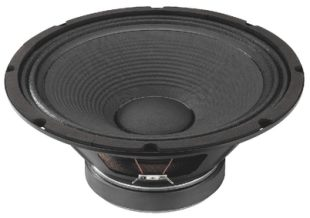recommended cabinet 1:
closed cabinet with 27 L volume
from 96/61 Hz (-3dB/-8dB)

recommended cabinet 2:
110 L volume bass reflex cabinet
with 2x HP100 reflex tube, 6 cm long.
from 46/38 Hz (-3dB/-8dB).

SP-12/100PA       order no. mo-102510       - not available (_)
30 cm bass driver with high quality cone.
• power handling (continuous/programme) = 100/200 W
• frequency range = 60-5000 Hz
• resonance frequency fs = 55 Hz
• impedance R = 8 Ohm
• sound pressure level SPL = 100 dB (2,83V; 1m)
• DC resistance Re = 6,8 Ohm
• force factor BL = 14,1 N/A
• voice coil inductance L = 0,8 mH
• effective piston radiating area Sd = 531 cm2
• effective mechanical mass incl. air load mms = 43 g
• equivalent volume of compliance Vas = 68 l
• total Q factor Qts = 0,46 (Qms=4,34, Qes=0,51)
• voice coil diameter = 50 mm
• mounting diameter d = 280 mm
• overall diameter d = 310 mm
• mounting depth (not countersunk) t = 125 mmrecommended cabinet 1:
closed cabinet with 25 L volume
from 88/55 Hz (-3dB/-8dB)

recommended cabinet 2:
101 L volume bass reflex cabinet
with 2x HP100 reflex tube, 11 cm long.
from 42/35 Hz (-3dB/-8dB).

SPA-30PA       order no. mo-104040       - not available (_)
30 cm bass-midrange driver with paper cone. The basket is made of die-cast.
• power handling (continuous/programme) = 175/350 W
• frequency range = 50-5000 Hz
• resonance frequency fs = 49 Hz
• impedance R = 8 Ohm
• sound pressure level SPL = 98 dB (2,83V; 1m)
• DC resistance Re = 6 Ohm
• force factor BL = 13,42 N/A
• voice coil inductance L = 0,87 mH
• effective piston radiating area Sd = 490 cm2
• effective mechanical mass incl. air load mms = 52 g
• equivalent volume of compliance Vas = 67 l
• total Q factor Qts = 0,45 (Qms=2,71, Qes=0,54)
• voice coil diameter = 50 mm
• mounting diameter d = 284 mm
• overall diameter d = 318 mm
• mounting depth (not countersunk) t = 124 mmrecommended cabinet 1:
closed cabinet with 6,3 L volume
from 168/106 Hz (-3dB/-8dB)

recommended cabinet 2:
15 L volume bass reflex cabinet
with HP100 reflex tube, 14 cm long.
from 100/75 Hz (-3dB/-8dB).

SP-12A/300NEO       order no. mo-104610       - not available (_)
30 cm bass driver with high quality cone. The magnet system is based on Neodymium technology. The basket is made of die-cast.
• power handling (continuous/programme) = 300/600 W
• frequency range = 30-3500 Hz
• resonance frequency fs = 53 Hz
• impedance R = 8 Ohm
• sound pressure level SPL = 103 dB (2,83V; 1m)
• DC resistance Re = 5,5 Ohm
• force factor BL = 17,8 N/A
• voice coil inductance L = 0,3 mH
• effective piston radiating area Sd = 500 cm2
• effective mechanical mass incl. air load mms = 45 g
• equivalent volume of compliance Vas = 68 l
• total Q factor Qts = 0,25 (Qms=7,93, Qes=0,26)
• voice coil diameter = 65 mm
• mounting diameter d = 281 mm
• overall diameter d = 312 mm
• mounting depth (not countersunk) t = 116 mmopen a bigger photo

recommended cabinet 1:
closed cabinet with 24 L volume
from 86/54 Hz (-3dB/-8dB)

recommended cabinet 2:
94 L volume bass reflex cabinet
with 2x HP100 reflex tube, 14 cm long.
from 41/34 Hz (-3dB/-8dB).

SPA-12PA       order no. mo-105280       - not available (_)
30 cm bass-midrange driver with high quality cone. The basket is made of die-cast. To avoid compression effects the driver possesses a pole piece hole.
• power handling (continuous/programme) = 150/300 W
• frequency range = f3-3500 Hz
• resonance frequency fs = 47 Hz
• impedance R = 8 Ohm
• sound pressure level SPL = 96 dB (2,83V; 1m)
• DC resistance Re = 6,3 Ohm
• force factor BL = 15,75 N/A
• voice coil inductance L = 1,1 mH
• effective piston radiating area Sd = 530 cm2
• effective mechanical mass incl. air load mms = 67 g
• equivalent volume of compliance Vas = 67 l
• total Q factor Qts = 0,43 (Qms=3,33, Qes=0,50)
• voice coil diameter = 63 mm
• mounting diameter d = 282 mm
• overall diameter d = 310 mm
• mounting depth (not countersunk) t = 130 mm
• mass m = 4,5 kg

## 15 inch wooferrecommended cabinet 1:
closed cabinet with 11,8 L volume
from 155/98 Hz (-3dB/-8dB)

recommended cabinet 2:
29 L volume bass reflex cabinet
with 2x HP100 reflex tube, 15 cm long.
from 93/70 Hz (-3dB/-8dB).

SP-15A/350NEO       order no. mo-104620       - not available (_)
37 cm bass driver with high quality cone. The magnet system is based on Neodymium technology. The basket is made of die-cast.
• power handling (continuous/programme) = 350/700 W
• frequency range = 60-3000 Hz
• resonance frequency fs = 49 Hz
• impedance R = 8 Ohm
• sound pressure level SPL = 105 dB (2,83V; 1m)
• DC resistance Re = 5,5 Ohm
• force factor BL = 22,5 N/A
• voice coil inductance L = 0,7 mH
• effective piston radiating area Sd = 840 cm2
• effective mechanical mass incl. air load mms = 73 g
• equivalent volume of compliance Vas = 128 l
• total Q factor Qts = 0,25 (Qms=7,75, Qes=0,26)
• voice coil diameter = 75 mm
• mounting diameter d = 350 mm
• overall diameter d = 390 mm
• mounting depth (not countersunk) t = 141 mmrecommended cabinet 1:
closed cabinet with 38 L volume
from 90/57 Hz (-3dB/-8dB)

recommended cabinet 2:
117 L volume bass reflex cabinet
with 2x HP100 reflex tube, 8 cm long.
from 48/38 Hz (-3dB/-8dB).

SP-15A/402PA       order no. mo-104940       EUR 282,60excl. VAT: € 237.48 / \$ 256.48
37 cm bass driver with high quality cone. The basket is made of die-cast. To avoid compression effects the driver possesses a pole piece hole.
• power handling (continuous/programme) = 400/800 W
• frequency range = 40-3000 Hz
• resonance frequency fs = 36 Hz
• impedance R = 8 Ohm
• sound pressure level SPL = 100 dB (2,83V; 1m)
• DC resistance Re = 6,5 Ohm
• force factor BL = 19,9 N/A
• voice coil inductance L = 0,9 mH
• effective piston radiating area Sd = 881 cm2
• effective mechanical mass incl. air load mms = 87 g
• equivalent volume of compliance Vas = 235 l
• total Q factor Qts = 0,31 (Qms=7,45, Qes=0,33)
• voice coil diameter = 100 mm
• mounting diameter d = 350 mm
• overall diameter d = 390 mm
• mounting depth (not countersunk) t = 145 mmSP-384PA       order no. mo-103960       EUR 121,70excl. VAT: € 102.27 / \$ 110.45
37 cm bass driver with high quality cone.
• power handling (continuous/programme) = 250/500 W
• frequency range = 40-3500 Hz
• resonance frequency fs = 38 Hz
• impedance R = 4 Ohm
• sound pressure level SPL = 96 dB (2,83V; 1m)
• mounting diameter d = 353 mm
• overall diameter d = 390 mm
• mounting depth (not countersunk) t = 149 mmSP-380PA       order no. mo-102990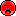- not available
37 cm bass driver with high quality cone.
• power handling (continuous/programme) = 250/500 W
• frequency range = 40-3500 Hz
• resonance frequency fs = 38 Hz
• impedance R = 8 Ohm
• sound pressure level SPL = 96 dB (2,83V; 1m)
• mounting diameter d = 353 mm
• overall diameter d = 390 mm
• mounting depth (not countersunk) t = 149 mmrecommended cabinet 1:
closed cabinet with 88 L volume
from 67/43 Hz (-3dB/-8dB)

recommended cabinet 2:
344 L volume bass reflex cabinet
with 2x HP100 reflex tube, 1 cm long.
from 33/27 Hz (-3dB/-8dB).

SP-38/300NEO       order no. mo-105110       EUR 216,10excl. VAT: € 181.60 / \$ 196.12
37 cm bass driver with high quality cone. The magnet system is based on Neodymium technology.
• power handling (continuous/programme) = 300/600 W
• frequency range = 40-4000 Hz
• resonance frequency fs = 36 Hz
• impedance R = 8 Ohm
• sound pressure level SPL = 101 dB (2,83V; 1m)
• DC resistance Re = 5,4 Ohm
• force factor BL = 13,5 N/A
• voice coil inductance L = 0,75 mH
• effective piston radiating area Sd = 880 cm2
• effective mechanical mass incl. air load mms = 71 g
• equivalent volume of compliance Vas = 265 l
• total Q factor Qts = 0,43 (Qms=4,13, Qes=0,48)
• voice coil diameter = 63 mm
• mounting diameter d = 354 mm
• overall diameter d = 388 mm
• mounting depth (not countersunk) t = 152 mm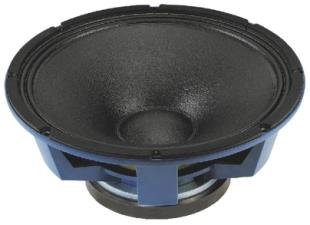recommended cabinet 1:
closed cabinet with 15 L volume
from 172/109 Hz (-3dB/-8dB)

recommended cabinet 2:
32 L volume bass reflex cabinet
with 2x HP100 reflex tube, 9 cm long.
from 108/81 Hz (-3dB/-8dB).

SP-38/300PA       order no. mo-103380       EUR 249,80excl. VAT: € 209.92 / \$ 226.71
37 cm bass driver with high quality cone.
• power handling (continuous/programme) = 300/600 W
• frequency range = 50-4000 Hz
• resonance frequency fs = 48 Hz
• impedance R = 8 Ohm
• sound pressure level SPL = 97 dB (2,83V; 1m)
• DC resistance Re = 5,9 Ohm
• force factor BL = 22 N/A
• voice coil inductance L = 1,2 mH
• effective piston radiating area Sd = 855 cm2
• effective mechanical mass incl. air load mms = 78 g
• equivalent volume of compliance Vas = 213 l
• total Q factor Qts = 0,23 (Qms=4,02, Qes=0,24)
• voice coil diameter = 76 mm
• mounting diameter d = 357 mm
• overall diameter d = 390 mm
• mounting depth (not countersunk) t = 158 mmrecommended cabinet 1:
closed cabinet with 33 L volume
from 85/53 Hz (-3dB/-8dB)

recommended cabinet 2:
112 L volume bass reflex cabinet
with 2x HP100 reflex tube, 11 cm long.
from 44/35 Hz (-3dB/-8dB).

SP-38A/500BS       order no. mo-104090       EUR 367,00excl. VAT: € 308.40 / \$ 333.08
37 cm bass driver with high quality cone.
• power handling (continuous/programme) = 500/1500 W
• frequency range = 50-3500 Hz
• resonance frequency fs = 37 Hz
• impedance R = 8 Ohm
• sound pressure level SPL = 97 dB (2,83V; 1m)
• DC resistance Re = 6 Ohm
• force factor BL = 18,25 N/A
• voice coil inductance L = 1,6 mH
• effective piston radiating area Sd = 855 cm2
• effective mechanical mass incl. air load mms = 107 g
• equivalent volume of compliance Vas = 168 l
• total Q factor Qts = 0,35 (Qms=4,37, Qes=0,38)
• voice coil diameter = 100 mm
• mounting diameter d = 355 mm
• overall diameter d = 385 mm
• mounting depth (not countersunk) t = 146 mmrecommended cabinet 1:
closed cabinet with 35 L volume
from 103/65 Hz (-3dB/-8dB)

recommended cabinet 2:
128 L volume bass reflex cabinet
with 2x HP100 reflex tube, 3 cm long.
from 52/41 Hz (-3dB/-8dB).

SP-38A/300PRO       order no. mo-104530       - not available (_)
37 cm bass driver with high quality cone. The basket is made of die-cast.
• power handling (continuous/programme) = 300/600 W
• frequency range = 70-4000 Hz
• resonance frequency fs = 50 Hz
• impedance R = 8 Ohm
• sound pressure level SPL = 102 dB (2,83V; 1m)
• DC resistance Re = 6 Ohm
• force factor BL = 18,54 N/A
• voice coil inductance L = 0,85 mH
• effective piston radiating area Sd = 880 cm2
• effective mechanical mass incl. air load mms = 80 g
• equivalent volume of compliance Vas = 135 l
• total Q factor Qts = 0,39 (Qms=3,37, Qes=0,44)
• voice coil diameter = 75 mm
• mounting diameter d = 354 mm
• overall diameter d = 392 mm
• mounting depth (not countersunk) t = 149 mmopen a bigger photo

recommended cabinet 1:
closed cabinet with 28 L volume
from 106/67 Hz (-3dB/-8dB)

recommended cabinet 2:
85 L volume bass reflex cabinet
with 2x HP100 reflex tube, 8 cm long.
from 58/44 Hz (-3dB/-8dB).

TRUVOX-1530       order no. mo-105010       - not available (_)
37 cm bass driver with cellulose cone.
• power handling (continuous/programme) = 400/800 W
• frequency range = 60-3000 Hz
• resonance frequency fs = 41 Hz
• impedance R = 8 Ohm
• sound pressure level SPL = 101 dB (2,83V; 1m)
• DC resistance Re = 5,9 Ohm
• force factor BL = 19,48 N/A
• voice coil inductance L = 0,9 mH
• effective piston radiating area Sd = 855 cm2
• effective mechanical mass incl. air load mms = 82 g
• equivalent volume of compliance Vas = 188 l
• total Q factor Qts = 0,31 (Qms=4,49, Qes=0,33)
• voice coil diameter = 75 mm
• mounting diameter d = 353 mm
• overall diameter d = 385 mm
• mounting depth (not countersunk) t = 162 mm
• mass m = 2,4 kgopen a bigger photo

recommended cabinet 1:
closed cabinet with 93 L volume
from 71/45 Hz (-3dB/-8dB)

recommended cabinet 2:
381 L volume bass reflex cabinet
with 2x HP100 reflex tube, -1 cm long.
from 33/28 Hz (-3dB/-8dB).

TRUVOX-1525       order no. mo-105000       - not available (_)
37 cm bass driver with cellulose cone.
• power handling (continuous/programme) = 250/500 W
• frequency range = 67-3000 Hz
• resonance frequency fs = 45 Hz
• impedance R = 8 Ohm
• sound pressure level SPL = 100 dB (2,83V; 1m)
• DC resistance Re = 5,2 Ohm
• force factor BL = 14,18 N/A
• voice coil inductance L = 0,8 mH
• effective piston radiating area Sd = 855 cm2
• effective mechanical mass incl. air load mms = 73 g
• equivalent volume of compliance Vas = 168 l
• total Q factor Qts = 0,5 (Qms=5,55, Qes=0,55)
• voice coil diameter = 63 mm
• mounting diameter d = 354 mm
• overall diameter d = 385 mm
• mounting depth (not countersunk) t = 152 mm
• mass m = 4,3 kgopen a bigger photo

recommended cabinet 1:
closed cabinet with 121 L volume
from 58/37 Hz (-3dB/-8dB)

recommended cabinet 2:
479 L volume bass reflex cabinet
with 2x HP100 reflex tube, 0 cm long.
from 26/23 Hz (-3dB/-8dB).

SP-15PA       order no. mo-105290       - not available (_)
37 cm bass driver with high quality cone. The basket is made of die-cast. To avoid compression effects the driver possesses a pole piece hole.
• power handling (continuous/programme) = 200/350 W
• frequency range = 60-2500 Hz
• resonance frequency fs = 41 Hz
• impedance R = 8 Ohm
• sound pressure level SPL = 98 dB (2,83V; 1m)
• DC resistance Re = 6 Ohm
• force factor BL = 16,64 N/A
• voice coil inductance L = 1,5 mH
• effective piston radiating area Sd = 855 cm2
• effective mechanical mass incl. air load mms = 102 g
• equivalent volume of compliance Vas = 147 l
• total Q factor Qts = 0,56 (Qms=13,62, Qes=0,58)
• voice coil diameter = 75 mm
• mounting diameter d = 352 mm
• overall diameter d = 389 mm
• mounting depth (not countersunk) t = 157 mm
• mass m = 5,8 kg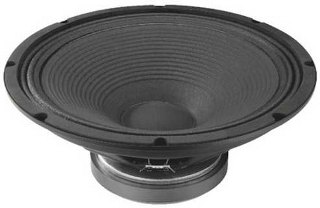open a bigger photo

recommended cabinet 1:
closed cabinet with 27 L volume
from 108/68 Hz (-3dB/-8dB)

recommended cabinet 2:
75 L volume bass reflex cabinet
with 2x HP100 reflex tube, 10 cm long.
from 61/47 Hz (-3dB/-8dB).

SP-15/300PA       order no. mo-102540       - not available (_)
37 cm bass driver with high quality cone.
• power handling (continuous/programme) = 300/600 W
• frequency range = 57-4500 Hz
• resonance frequency fs = 38 Hz
• impedance R = 8 Ohm
• sound pressure level SPL = 101 dB (2,83V; 1m)
• DC resistance Re = 6,8 Ohm
• force factor BL = 22,27 N/A
• voice coil inductance L = 1,3 mH
• effective piston radiating area Sd = 855 cm2
• effective mechanical mass incl. air load mms = 88 g
• equivalent volume of compliance Vas = 232 l
• total Q factor Qts = 0,28 (Qms=5,44, Qes=0,29)
• voice coil diameter = 78 mm
• mounting diameter d = 358 mm
• overall diameter d = 388 mm
• mounting depth (not countersunk) t = 156 mm
• mass m = 7 kgopen a bigger photo

recommended cabinet 1:
closed cabinet with 13,4 L volume
from 107/68 Hz (-3dB/-8dB)

recommended cabinet 2:
28 L volume bass reflex cabinet
with HP100 reflex tube, 21 cm long.
from 68/50 Hz (-3dB/-8dB).

FTR15-4080F       order no. mo-105050       - not available (_)
37 cm bass driver with high quality cone. To avoid compression effects the driver possesses a pole piece hole. The spider is rear ventilated for better cooling.
• power handling (continuous/programme) = 600/1200 W
• frequency range = 45-1500 Hz
• resonance frequency fs = 30 Hz
• impedance R = 8 Ohm
• sound pressure level SPL = 98 dB (2,83V; 1m)
• DC resistance Re = 5,5 Ohm
• force factor BL = 25,81 N/A
• voice coil inductance L = 1,5 mH
• effective piston radiating area Sd = 855 cm2
• effective mechanical mass incl. air load mms = 150 g
• equivalent volume of compliance Vas = 190 l
• total Q factor Qts = 0,22 (Qms=3,41, Qes=0,24)
• voice coil diameter = 100 mm
• mounting diameter d = 352 mm
• overall diameter d = 388 mm
• mounting depth (not countersunk) t = 175 mm
• mass m = 9,4 kgrecommended cabinet 1:
closed cabinet with 93 L volume
from 65/41 Hz (-3dB/-8dB)

recommended cabinet 2:
372 L volume bass reflex cabinet
with 2x HP100 reflex tube, 1 cm long.
from 31/25 Hz (-3dB/-8dB).

SPA-38PA       order no. mo-104050       - not available (_)
38 cm bass driver with paper cone. The basket is made of die-cast.
• power handling (continuous/programme) = 250/500 W
• frequency range = 55-3500 Hz
• resonance frequency fs = 37 Hz
• impedance R = 8 Ohm
• sound pressure level SPL = 98 dB (2,83V; 1m)
• DC resistance Re = 6 Ohm
• force factor BL = 14,32 N/A
• voice coil inductance L = 1 mH
• effective piston radiating area Sd = 855 cm2
• effective mechanical mass incl. air load mms = 80 g
• equivalent volume of compliance Vas = 230 l
• total Q factor Qts = 0,45 (Qms=2,54, Qes=0,55)
• voice coil diameter = 65 mm
• mounting diameter d = 354 mm
• overall diameter d = 402 mm
• mounting depth (not countersunk) t = 138 mm

## 18 inch wooferSP-450PA       order no. mo-103000       - not available (_)
44 cm bass driver with high quality cone.
• power handling (continuous/programme) = 300/600 W
• frequency range = 37-3500 Hz
• resonance frequency fs = 25 Hz
• impedance R = 8 Ohm
• sound pressure level SPL = 97 dB (2,83V; 1m)
• mounting diameter d = 420 mm
• overall diameter d = 460 mm
• mounting depth (not countersunk) t = 196 mmrecommended cabinet 1:
closed cabinet with 36 L volume
from 88/56 Hz (-3dB/-8dB)

recommended cabinet 2:
96 L volume bass reflex cabinet
with 4x HP100 reflex tube, 39 cm long.
from 51/39 Hz (-3dB/-8dB).

SP-46/500PA       order no. mo-103410- not available
44 cm bass driver with paper cone.
• power handling (continuous/programme) = 500/1000 W
• frequency range = 45-2500 Hz
• resonance frequency fs = 30 Hz
• impedance R = 8 Ohm
• sound pressure level SPL = 101 dB (2,83V; 1m)
• DC resistance Re = 5,7 Ohm
• force factor BL = 25 N/A
• voice coil inductance L = 1,45 mH
• effective piston radiating area Sd = 1180 cm2
• effective mechanical mass incl. air load mms = 160 g
• equivalent volume of compliance Vas = 333 l
• total Q factor Qts = 0,27 (Qms=8,08, Qes=0,28)
• voice coil diameter = 100 mm
• mounting diameter d = 415 mm
• overall diameter d = 460 mm
• mounting depth (not countersunk) t = 185 mm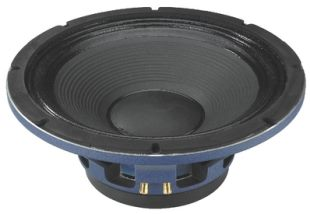recommended cabinet 1:
closed cabinet with 122 L volume
from 59/37 Hz (-3dB/-8dB)

recommended cabinet 2:
497 L volume bass reflex cabinet
with 4x HP100 reflex tube, 7 cm long.
from 28/23 Hz (-3dB/-8dB).

SP-46A/500BS       order no. mo-104100       EUR 384,80excl. VAT: € 323.36 / \$ 349.23
45 cm bass driver with high quality cone.
• power handling (continuous/programme) = 500/2000 W
• frequency range = 54-1800 Hz
• resonance frequency fs = 36 Hz
• impedance R = 8 Ohm
• sound pressure level SPL = 98 dB (2,83V; 1m)
• DC resistance Re = 6 Ohm
• force factor BL = 17,49 N/A
• voice coil inductance L = 1,65 mH
• effective piston radiating area Sd = 1163 cm2
• effective mechanical mass incl. air load mms = 141 g
• equivalent volume of compliance Vas = 250 l
• total Q factor Qts = 0,48 (Qms=4,75, Qes=0,54)
• voice coil diameter = 100 mm
• mounting diameter d = 420 mm
• overall diameter d = 470 mm
• mounting depth (not countersunk) t = 189 mm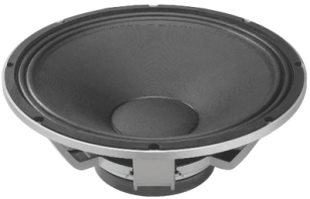recommended cabinet 1:
closed cabinet with 177 L volume
from 51/32 Hz (-3dB/-8dB)

recommended cabinet 2:
712 L volume bass reflex cabinet
with 4x HP100 reflex tube, 6 cm long.
from 24/20 Hz (-3dB/-8dB).

SPA-45PA       order no. mo-104060       - not available (_)
45 cm bass driver with paper cone. The basket is made of die-cast.
• power handling (continuous/programme) = 300/600 W
• frequency range = 44-3500 Hz
• resonance frequency fs = 29 Hz
• impedance R = 8 Ohm
• sound pressure level SPL = 98 dB (2,83V; 1m)
• DC resistance Re = 5,8 Ohm
• force factor BL = 16,91 N/A
• voice coil inductance L = 1 mH
• effective piston radiating area Sd = 1225 cm2
• effective mechanical mass incl. air load mms = 136 g
• equivalent volume of compliance Vas = 440 l
• total Q factor Qts = 0,46 (Qms=3,77, Qes=0,52)
• voice coil diameter = 75 mm
• mounting diameter d = 424 mm
• overall diameter d = 464 mm
• mounting depth (not countersunk) t = 159 mm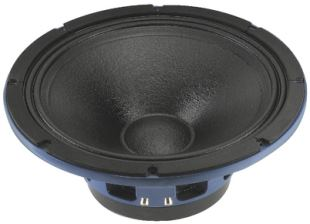recommended cabinet 1:
closed cabinet with 26 L volume
from 101/64 Hz (-3dB/-8dB)

recommended cabinet 2:
64 L volume bass reflex cabinet
with 2x HP100 reflex tube, 16 cm long.
from 60/45 Hz (-3dB/-8dB).

SP-46A/500PA       order no. mo-103420       - not available (_)
45 cm bass driver with paper cone. The basket is made of die-cast.
• power handling (continuous/programme) = 500/2000 W
• frequency range = 48-2500 Hz
• resonance frequency fs = 32 Hz
• impedance R = 8 Ohm
• sound pressure level SPL = 99 dB (2,83V; 1m)
• DC resistance Re = 6,4 Ohm
• force factor BL = 29,1 N/A
• voice coil inductance L = 1,7 mH
• effective piston radiating area Sd = 1180 cm2
• effective mechanical mass incl. air load mms = 168 g
• equivalent volume of compliance Vas = 285 l
• total Q factor Qts = 0,25 (Qms=6,65, Qes=0,26)
• voice coil diameter = 99 mm
• mounting diameter d = 416 mm
• overall diameter d = 470 mm
• mounting depth (not countersunk) t = 194 mmopen a bigger photo

recommended cabinet 1:
closed cabinet with 36 L volume
from 82/52 Hz (-3dB/-8dB)

recommended cabinet 2:
85 L volume bass reflex cabinet
with 2x HP100 reflex tube, 20 cm long.
from 50/37 Hz (-3dB/-8dB).

FTR18-4080F       order no. mo-105060       - not available (_)
44 cm bass driver with high quality cone. To avoid compression effects the driver possesses a pole piece hole. The spider is rear ventilated for better cooling.
• power handling (continuous/programme) = 600-1200 W
• frequency range = 37-500 Hz
• resonance frequency fs = 25 Hz
• impedance R = 8 Ohm
• sound pressure level SPL = 99 dB (2,83V; 1m)
• DC resistance Re = 5,6 Ohm
• force factor BL = 24,12 N/A
• voice coil inductance L = 1,5 mH
• effective piston radiating area Sd = 1164 cm2
• effective mechanical mass incl. air load mms = 169 g
• equivalent volume of compliance Vas = 430 l
• total Q factor Qts = 0,24 (Qms=3,78, Qes=0,26)
• voice coil diameter = 100 mm
• mounting diameter d = 414 mm
• overall diameter d = 453 mm
• mounting depth (not countersunk) t = 206 mm
• mass m = 9,7 kg
 Tweeter Mids Fullrange Woofer no longer available PA Mids Woofer Fullrange no longer available Car Woofer no longer available
The cabinet volume calculations are executed considering an external resistance of 0.3 Ohm. We are using, if no other data is known or available, the information supplied by the manufacturer.

Please note: the frequency response doesn't say much about the cut-off frequency inside the cabinet! Therefore, we mostly give you 2 examples with dimensioning considerations and an indication, how low the loudspeaker will reproduce.

Home